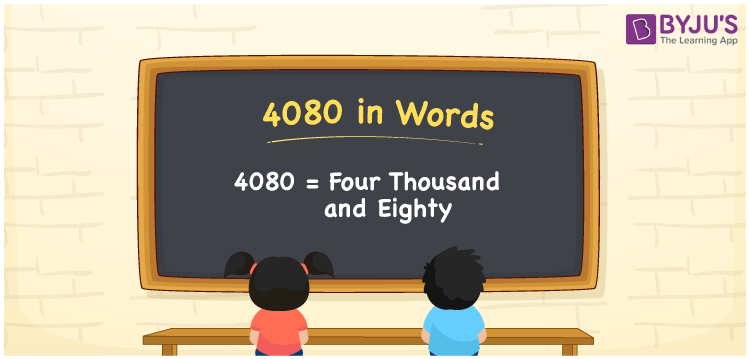# 4080 in Words

The number 4080 in words is written as Four thousand eighty. In both the International and Indian System of Numerals, 4080 is written as 4,080 and read as Four thousand eighty. We may use 4080 as “that gadget costs 4080 rupees” in this context, 4080 represents an amount; that is why 4080 is a Cardinal Number since it represents some quantity.

 4080 in Words Four Thousand Eighty Four Thousand Eighty in Numbers 4080

## 4080 in English Words

In English words, 4080 is written as “Four thousand eighty”.## How to Write 4080 in Words?

To write 4080 in words, we shall use the place value. In the place value chart, write 4 in thousands, 0 in hundreds, 8 in tens and 0 in ones. Let us make a place value chart to write the number 4080 in words.

 Thousands Hundreds Tens Ones 4 0 8 0

Thus, we can the expanded form as

4 × Thousand + 0 × Hundred + 8 × Ten + 0 × One

= 4 × 1000 + 0 × 100 + 8 × 10 + 0 × 1

= 4000 + 0 + 80 + 0

= 4080

= Four thousand eighty.

4080 is a Natural Number, the successor of 4079 and the predecessor of 4081

4080 in words – Four thousand eighty

• Is 4080 an odd number? – No
• Is 4080 an even number? – Yes
• Is 4080 a perfect square number? – No
• Is 4080 a perfect cube number? – No
• Is 4080 a prime number? – No
• Is 4080 a composite number? – Yes

## Frequently Asked Questions on 4080 in Words

Q1

### How to write 4080 in words?

4080 in words is written as Four thousand eighty.
Q2

### How to write 4080 in the International and Indian System of Numerals?

In both, the system of numerals, 4080 in words, is written as Four thousand eighty.
Q3

### How to write 4080 in a place value chart?

In the place value chart, write 4 in thousands, 0 in hundreds, 8 in tens and 0 in ones, respectively.